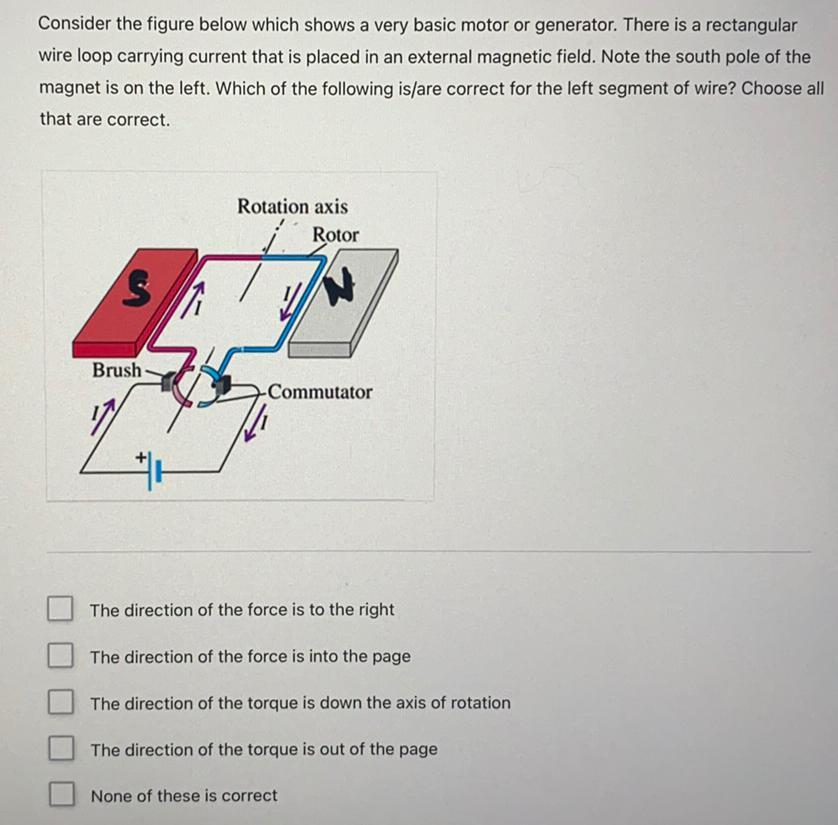Question:

# Consider the figure below which shows a very basic motor or generator. There is a rectangular wire loop carrying current that isConsider the figure below which shows a very basic motor or generator. There is a rectangular wire loop carrying current that is placed in an external magnetic field. Note the south pole of the magnet is on the left. Which of the following is/are correct for the left segment of wire? Choose all that are correct. Rotation axis Rotor S Brush Commutator The direction of the force is to the right The direction of the force is into the page The direction of the torque is down the axis of rotation The direction of the torque is out of the page None of these is correct